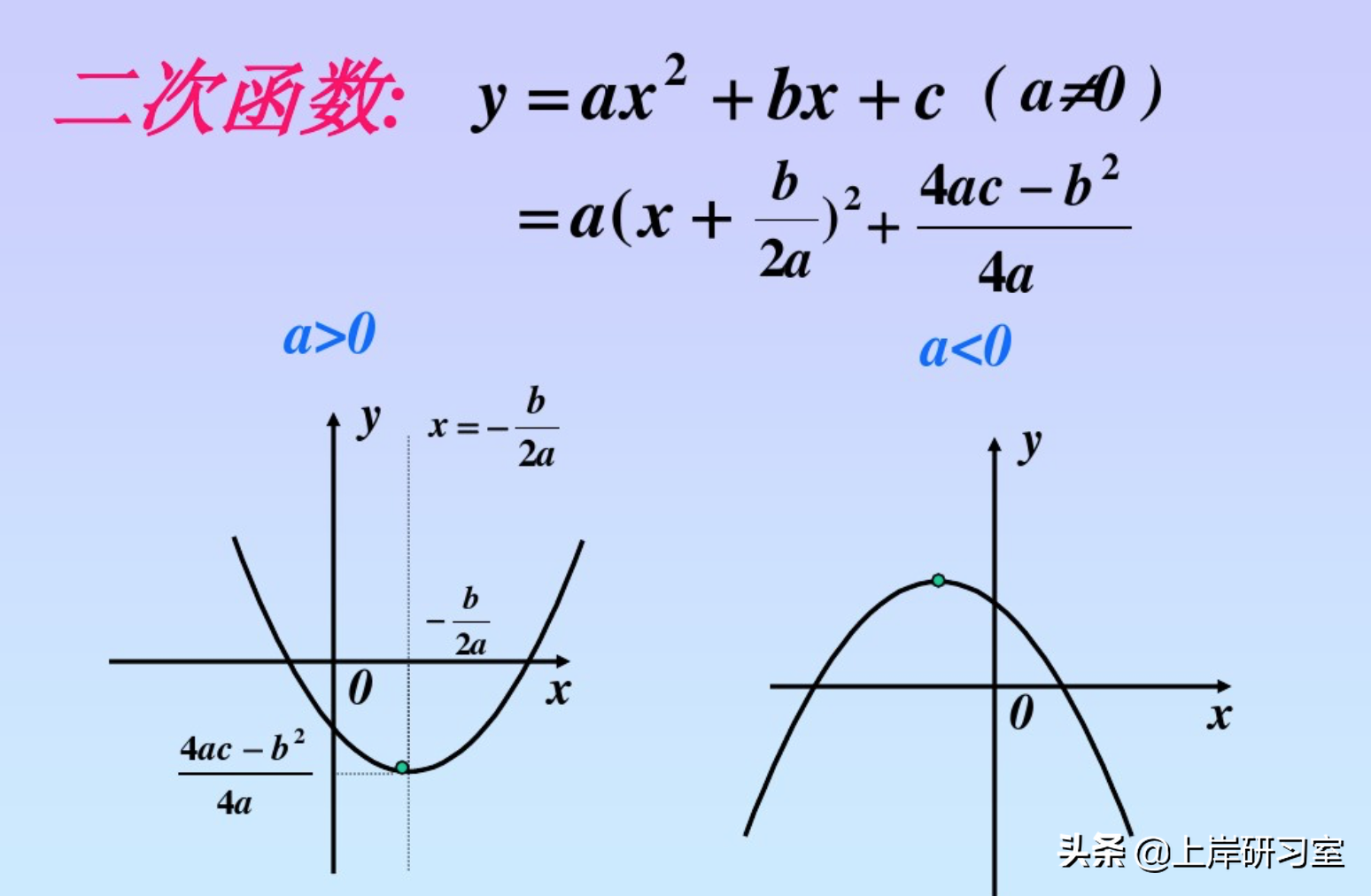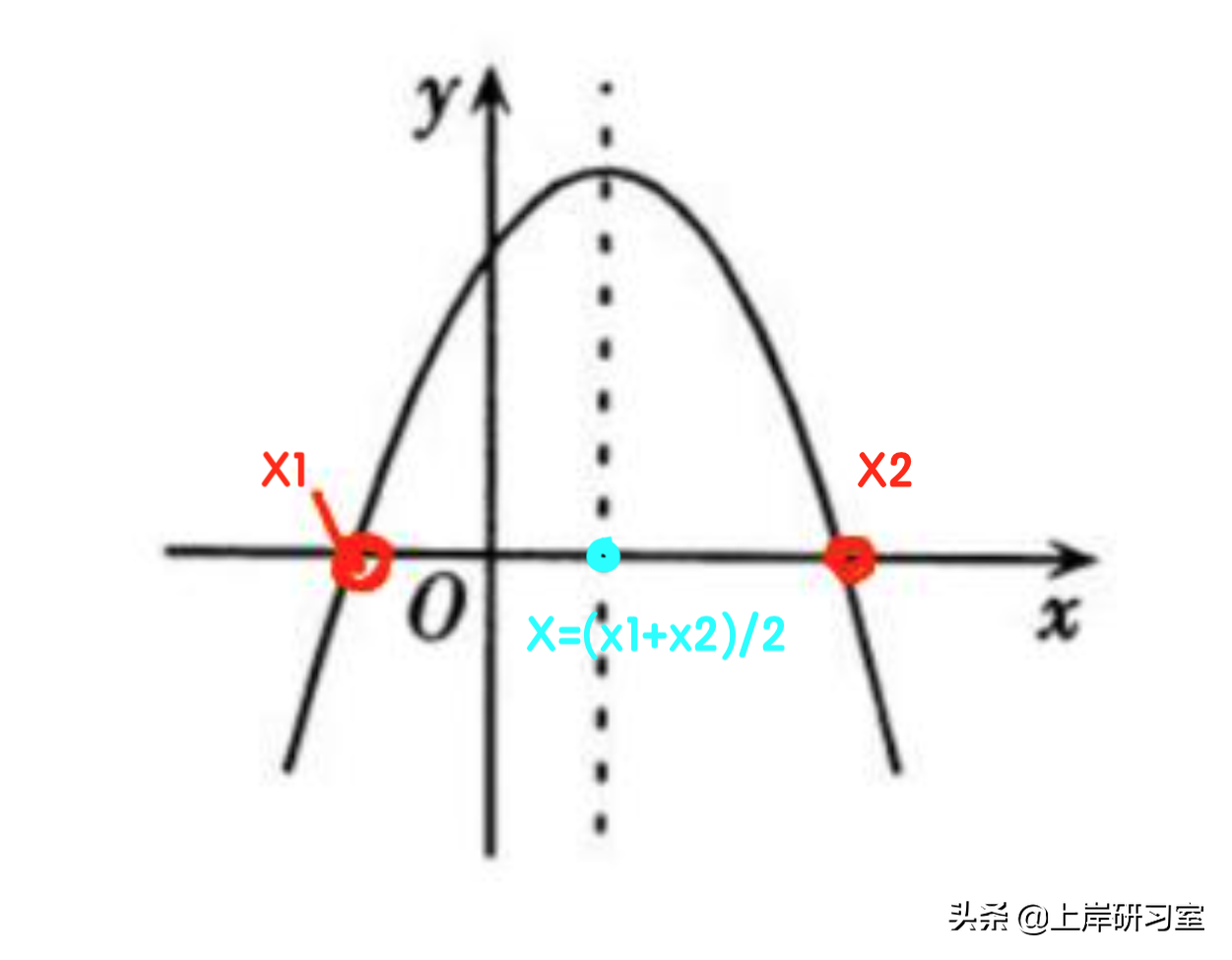# 二次函数最大值公式「建议收藏」

|本文有892个文字，大小约为4KB，预计阅读时间3分钟

函数最值问题的常见题型分两种，一种是二次函数型，一种是和一定型。今天先来看看二次函数型。

1.二次函数表达式为y=ax²+bx+c(且a≠0)，它是一个二次多项式(或单项式)。

2.题型特征:常以经济利润的模型相结合的方式进行考察。

①直接给出函数表达式求最值。

方法：y=ax²+bx+c 当x=-(b/2a)时，y 取得最值。

例一：某商业银行的总利润 P 与贷款数量 Q 之间的函数关 系为:P=10000+400Q-Q2，当贷款数量为( )万元时，总利润最大。

A.100 B.150 C.200 D.250

解析：

(1)题目中直接给出了函数关系P=10000+400Q-Q2 ，整理可得P=-Q2+400Q+10000。

(2)直接运用结论：当 Q=-b/2a→(-400)/(-1*2)=200 时，取得最大值，因此选择C选项。

直接给出函数关系的最值问题，直接带入x=-(b/2a)时，y 取得最值这一结论即可。二次函数最值图

②此消彼长，薄利多销型

这种题一般就是先给出一个方案，然后进行调整，常常会出现“每上升/下降....就多/少....”

例如：每涨价x元——销量下降y个。 每降价x元——销量上升y个。

方法：

1.根据条件和所求列方程，写成两括号相乘的形式。

2.求出使方程等于 0 时的两个根:x1、x2。

3.求出两个的平均值(对称轴):当 x=(x1+x2)/2 时，此时乘积最大。

例如：y =( 7 5 - x )*( 5 0 0 + 2 0 x )，使两个括号分别等于 0 ，即 7 5 - x = 0 → x1 = 7 5 ， 500 + 20x = → x2 = -25。求平均值(对称轴) x=(75-25)/2=25。∴当x = 25时，y取最大值。中轴处y取最大值

例二：某企业设计了一款工艺品，每件的成本是 70 元，为了 合理定价，投放市场进行试销。据市场调查，销售单价是 120 元时，每天的销售 量是 100 件，而销售单价每降价 1 元，每天就可多售出 5 件，但要求销售单价不 得低于成本。则销售单价为多少元时，每天的销售利润最大?

A.100 元 B.102 元 C.105 元 D.108 元

解析：

(1)根据总利润=单个商品利润 * 销量，商品成本为70，原单价为120，原单利为120-70=50。

(2)假设降价 x 次，即降价 x 元，利润为 50-x，销量为 100+5x，可列式 利润=(50-x)*(100+5x)。令 y=0，50-x=0→x1=50，100+5x=0→x2=-20，取中 x=(50-20)/2=15。

(3)x解得=15，即降价 15 次，共降 15 元，因此现单价=120-15=105 元。所以这道题选择C选项。

例三：某汽车坐垫加工厂生产一种汽车座垫，每套成本是 144 元，售价是 200 元。一个经销商订购了 120 套这种汽车座垫，并提出:如果每套 座垫的售价每降低 2 元，就多订购 6 套。按经销商的要求，该加工厂获得最大利 润需售出的套数是:

A.144 B.136 C.128 D.142

解析：

(1)根据题意成本为 144，售价为 200，利润为 200-144=56 元。

(2)假设降价 x 次，则降价 2x 元，销量增加 6x 套。利润 y=单利*销量=(56-2x)(120+6x)，令 y=0，56-2x=0→x1=56/2=28，120+6x=0→x2=-20，取中 x=(28-20)/2=4。

(3)降价 4 次，销量增加 6*4=24 套，总销量=120+24=144 套。因此这道题选择A选项。

※如果大家刷题多了之后，这道题根据倍数特性可以直接秒杀选项。

根据已知，销量=120+6x，即 销量-120 为 6 的倍数。因此，我们直接带入选项。

代入 A 项， 144-120=24 是 6 的倍数;

代入 B 项:136-120=16 不是 6 的倍数，C 项 128-120=8 不是 6 的倍数，D 项 142-120=22 不是 6 的倍数，只有 A 项满足。

在真正的考试中，只需带入A满足即可不用再考虑后边的选项。